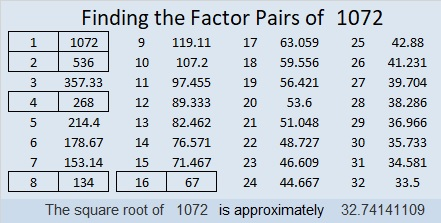# 1072 Find the Factors Challenge

These Find the Factors Challenge puzzles are tougher than my other puzzles, but they can still be solved using logic and basic multiplication and division facts. Go ahead, give it a try! I sincerely hope you will succeed!Print the puzzles or type the solution in this excel file: 12 factors 1063-1072

Now I’ll share some facts about the number 1072:

• 1072 is a composite number.
• Prime factorization: 1072 = 2 × 2 × 2 × 2 × 67, which can be written 1072 = 2⁴ × 67
• The exponents in the prime factorization are 4 and 1. Adding one to each and multiplying we get (4 + 1)(1 + 1) = 5 × 2 = 10. Therefore 1072 has exactly 10 factors.
• Factors of 1072: 1, 2, 4, 8, 16, 67, 134, 268, 536, 1072
• Factor pairs: 1072 = 1 × 1072, 2 × 536, 4 × 268, 8 × 134, or 16 × 67
• Taking the factor pair with the largest square number factor, we get √1072 = (√16)(√67) = 4√67 ≈ 32.741411072 is also palindrome 646 in BASE 13 because 6(13²) + 4(13) + 6(1) = 1072